Visitors Online: 77 | Monday 11th November 2019

CBSE Guess > Papers > Question Papers > Class XII > 2003 > Chemistry > Compartment Outside Delhi Set -I.

CHEMISTRY—2003 (Set I—Compartment Outside Delhi)

Q. 1. What is the state of hybridization of carbon in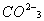ion? 1

Q. 2. How are the orbitalsoriented in space? 1

Q. 3. Name one solid in which both Frenkel and 'Schottky defects occur 1

Q. 4. How is it that the boiling points of the following solutions in water are different:
(a) 0.1 M NaCI solution
(b) 0.1 M sugar solution.

Q. 5. In the equation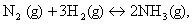what would be the sign of work done? 1

Q. 6. Why are the alkali metals not obtained by the chemical reduction methods? 1

Q. 7. An aqueous solution of ferric chloride is acidic. Give reason. 1

Q. 8. In what way is a sol different from a gel? 1

Q. 9. Why is bithional added to the toiled soap? 1

Q. 10. Give an example of a narcotic which is used as an analgesic. 1

Q. 11. Calculate the number of photons having a wavelength of 500 nm which would provide 1.2 J of energy. 2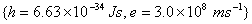Q. 12. The density of chromium metal is 7.2 g cm -3 . If the unit cell has edge length of 289 pm, determine the type of unit cell.
Atomic mass of chromium is 52 amu;2

Q. 13. Calculate the osmotic pressure of a solution obtained by mixing 100 cm 3 of 0.25 M solution of urea and 100 cm 3 of 0.1 M solution of one-sugar at 293 K.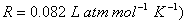Q. 14. 1.0 g of a non-volatile solute was dissolved in 100 g of acetone (mol. mass = 58 g) at 298 K. The vapour pressure of solution was found to be 192.5 mm of Hg. Calculate the molar mass of solute. (The vapour pressure of pure acetone at 298 K is 195 mm Hg] 2

Q. 15. Define the tern 'entropy'. Predict the sign of entropy change in each of the Following: 2
(a)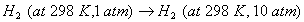(b)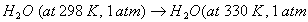(c)Q. 16. Calculate the standard free energy change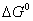for the reaction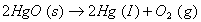Q. 17. Explain why electrolysis of aqueous solution of NaCI gives H 2 at cathode and CI 2 at anode. Write overall reaction.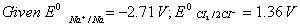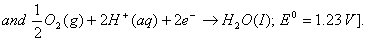Q. 18. Calculate the cell emf and AG for the cell reaction at 250C for the cell 2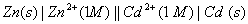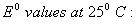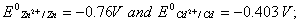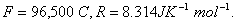Q. 19. Explain the term photosensitization giving an example. 2

Q. 20. The reaction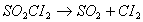is a first order reaction with k = 2.2 x 10 -5 s -1 at 575 K. What percentage ofS0 will get decomposed in 80 minutes, when the reaction is carried out at 575 K? 2

Q. 21. Give chemical tests to distinguish between the following pairs of compounds: 2
(a) Chloroform and Carbon tetrachloride
(b) Phenol and Benzoic acid

Q. 22. Account for the following:
(a) Ethers posses a dipole moments even if the alkyl radius in the molecule are identical.
(b) Sodium bisulphate is used for the purification of aldehydes and ketones.

Q. 23. Write the IUPAC names of the following compounds:
(i) C 6 H 5 N + (CH3) Br -
(ii) CH 3 (CH 2) 4 -CHCN
|
CH 3

Q. 24. In terms of their mode of formation how is chain growth polymerization different from step growth polymerization? Give one example of each. 2

Q. 25. Write two differences between lyophilic sols and lyophobic sols. Give one example of each.

Q. 26. What are antibodies? How do they fight a bacterium? 3

Q. 27. Explain the following with suitable examples:
(a) Kharasch effect
(b) Sandmeyer's reaction
(c) Kolbe-Schmidt reaction

Q. 28. How are the following obtained: 3
(a) 2-phenyl-2-butanol from acetophenone
(b) Lactic acid from acetylene
(c) Diethyl ether from ethene
Write reactions and conditions involved.

Q. 29. What happens when: (Write reactions only) 3
(a) Nitroethane Is treated with LiAIH 4 .
(b) Diazonium chloride reacts with phenol in basic medium.
(c) Methyl cyanide is treated with methyl magnesium bromide followed by hydrolysis.

Q. 30. Describe briefly the following: 3
(a) Preparation of ferrocene (chemical equation only)
(b) Structure of Zeise's salt
(c) Isomerism shown by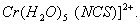Q. 31. (a) Why do thermonuclear reactions require very high temperature?
(b) One of the hazards of nuclear explosion is the generation of Sr-90 and its subsequent Incorporation in bones. This nuclide has a half-life period of 28.1 years. Suppose one microgram was absorbed by a newborn baby, how much Sr-90 will remain in his bones after 20 years? 3

Q. 32. Write four characteristic features of enzymes Name a disease which is caused by the deficiency of a particular enzyme. 3

Q. 33. Account for the following: 5
(a) Sn (II) is a reducing agent but Pb (II) is not.
(b) PH3 Is a weaker base than NH 3 .
(c) SF6 exists but SH 6 does not
(d) Sulphur exhibits tendency for catenation but oxygen does not
(e) The electron affinity of fluorine is less than that of chlorine.

Q. 34. (a) Explain the cause and consequences of lanthanide contraction.
(b) Give balanced chemical equations for the following reactions:
(i) Between acidified potassium dichromate and potassium Iodide.
(ii) Between potassium permanganate and oxalic acid In the presence of dilute sulphuric acid
(iii) Chromite ore is fused with NaOH in air.

 Chemistry 2003 Question Papers Class XII Delhi Outside Delhi Compartment Delhi Compartment Outside Delhi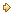Set ISet ISet ISet ISet IISet IISet IISet II

CBSE 2003 Question Papers Class XII